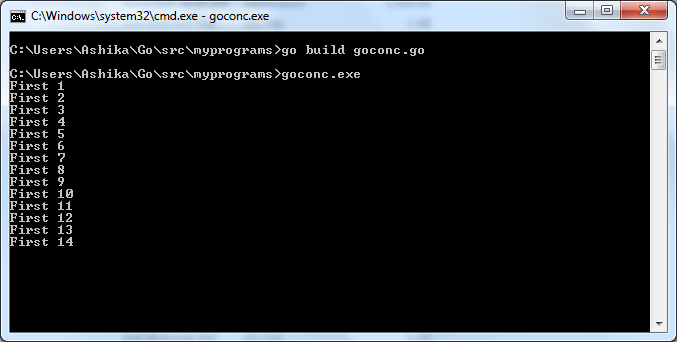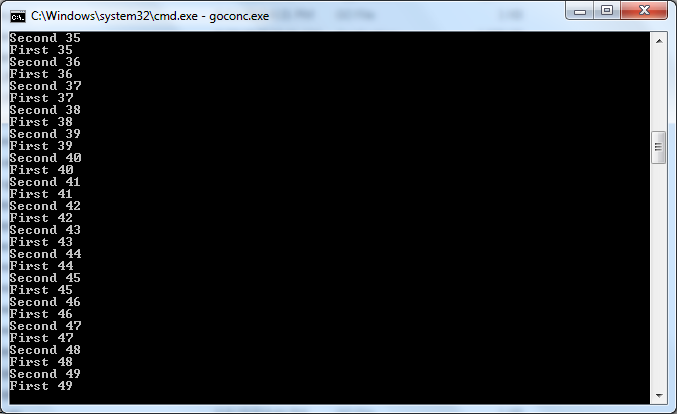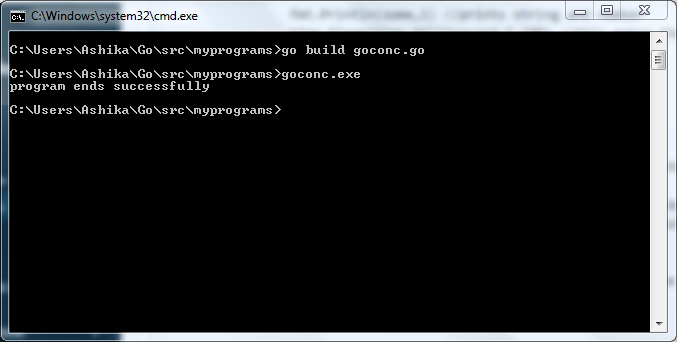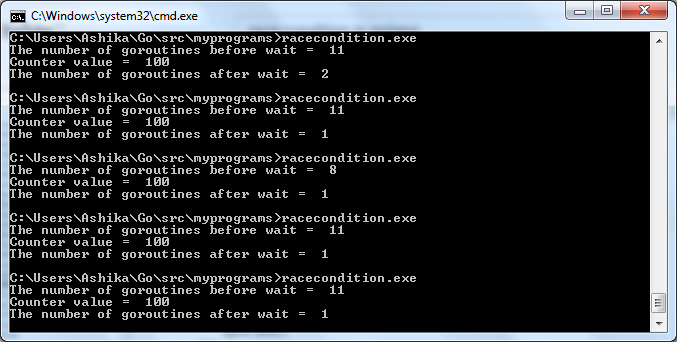GeeksforGeeks App
Open AppBrowser
Continue

As per Wikipedia, race condition is defined as the condition of an electronics, software, or other systems where the system’s substantive behavior is dependent on the sequence or timing of other uncontrollable events. Race condition falls under the “Concurrency” department. Concurrency is the art of making progress on multiple tasks simultaneously. Let’s understand what concurrency actually means.

Lets code and understand race condition practically!

 `package` `main`` ` `import` `(``    ``"fmt"``    ``"time"``)`` ` `func` `execute(some ``string``) {`` ` `    ``// initializing a infinite for loop``    ``for` `i := ``1``; true; i++ {`` ` `        ``// prints string and number``        ``fmt.Println(some, i)`` ` `        ``// this makes the program sleep for``        ``// 100 milliseconds, wiz 10 seconds``        ``time.Sleep(time.Millisecond * ``100``)`` ` `    ``}``}`` ` `func` `main() {`` ` `    ``// Simple go synchronous program ``    ``// without any concurrency``    ``execute(``"First"``)``     ` `    ``// when this func is called it executes``    ``// and then passes on to next line``    ``execute(``"Second"``)``     ` `    ``// after the first second is to be executed``    ``// the problem is, execute function will ``    ``// never finish execution, its trapped ``    ``// in an infinite loop so the program will ``    ``// never move to second execution.``    ``fmt.Println(``"program ends successfully"``)``     ` `    ``// if I'm wrong and both first and second ``    ``// execute, then this line should print too``    ``// check the output``}`If you use Windows, you’re likely to see such output on the screen. The above output goes on like that and has no end unless you manually stop it or it runs out of system memory for execution.

Consider the second code.

 `package` `main`` ` `import` `(``    ``"fmt"``    ``"time"``)`` ` `func` `execute(some ``string``) {`` ` `    ``// initializing a infinite for loop``    ``for` `i := ``1``; true; i++ {`` ` `        ``// prints string and number``        ``fmt.Println(some, i)`` ` `        ``// this makes the program sleep for``        ``// 100 milliseconds, wiz 10 seconds``        ``time.Sleep(time.Millisecond * ``100``)``    ``}``}`` ` `func` `main() {`` ` `    ``// Simple go program with concurrency``    ``go` `execute(``"First"``)`` ` `    ``// Placing the go command infront of the``    ``// func call simply creates a goroutine``    ``execute(``"Second"``)`` ` `    ``// The goroutine ensures that both functions``    ``// execute simultaneously & successfully``    ``fmt.Println(``"program ends successfully"``)`` ` `    ``// This statement still won't execute because``    ``// the func call is stuck in an infinite loop``    ``// check the output``}`If you use Windows, you’re likely to see such output on the screen. When you create a goroutine in Go, it typically makes the execution faster by giving off the waiting time to other prevailing tasks, Now let’s see what happens if you create two goroutines in the same program.

 `package` `main`` ` `import` `(``    ``"fmt"``    ``"time"``)`` ` `func` `execute(some ``string``) {`` ` `    ``// initializing a infinite for loop``    ``for` `i := ``1``; true; i++ {`` ` `        ``// prints string and number``        ``fmt.Println(some, i)``         ` `        ``// this makes the program sleep for``        ``// 100 milliseconds, wiz 10 seconds``        ``time.Sleep(time.Millisecond * ``100``) ``    ``}``}`` ` `func` `main() {`` ` `    ``// Simple go program with concurrency``    ``go` `execute(``"First"``)``     ` `    ``// Placing the go command in front of the``    ``// func call simply creates a goroutine``    ``go` `execute(``"Second"``)``     ` `    ``// The second goroutine, you may think that the``    ``// program will now run with lightning speed``    ``// But, both goroutines go to the background ``    ``// and result in no output at all Because the``    ``// program exits after the main goroutine``    ``fmt.Println(``"Program ends successfully"``)``     ` `    ``// This statement will now be executed``    ``// and nothing else will be executed``    ``// check the output``}`If you use Windows, you’re likely to see such output on the screen. Let’s consider one race condition scenario to wind up our topic:

 `package` `main`` ` `// one goroutine is the main``// goroutine that comes by default``import` `(``    ``"fmt"``    ``"runtime"``    ``"sync"``)`` ` `var` `wgIns sync.WaitGroup`` ` `func` `main() {`` ` `    ``// shared variable``    ``counter := ``0`` ` `    ``// the other 10 goroutines are``    ``// supposed to come from here``    ``wgIns.Add(``10``)``    ``for` `i := ``0``; i < ``10``; i++ {`` ` `        ``// goroutines are made``        ``go` `func``() {``            ``for` `j := ``0``; j < ``10``; j++ {`` ` `                ``// shared variable execution``                ``counter += ``1``                ``// 100 should be the counter value but``                ``// it may be equal to 100 or lesser``                ``// due to race condition``            ``}``            ``wgIns.Done()``        ``}()``    ``}`` ` `    ``// this value should actually be 11``    ``fmt.Println(``"The number of goroutines before wait = "``, runtime.NumGoroutine())`` ` `    ``wgIns.Wait()`` ` `    ``// value should be 100``    ``fmt.Println(``"Counter value = "``, counter)`` ` `    ``fmt.Println(``"The number of goroutines after wait = "``, runtime.NumGoroutine())`` ` `    ``// this value is supposed to be 1``    ``// but lets see if these values``    ``// stay consistently same every``    ``// time we run the code``}`This inconsistency happens because of race conditions. Race condition in simple terms can be explained as, you have one candy and two kids run to you claiming that they’re both hungry. They both end up fighting for that one chocolate, they race to get the candy. This is a race condition. Here the solution is: get another candy so that the two enjoy a candy peacefully. Likewise, we can increase the resources allocation in order to ensure race condition does not occur.

My Personal Notes arrow_drop_up